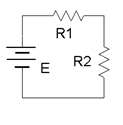# 9.4: Schematics and DiagramsFigure $$\PageIndex{1a}$$Figure $$\PageIndex{1b}$$Figure $$\PageIndex{2}$$